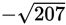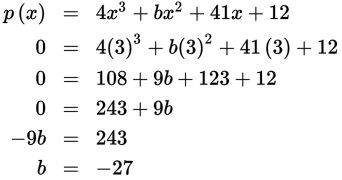# SAT Math Multiple Choice Question 921: Answer and Explanation

### Test Information

Question: 921

5. The polynomial function p is defined by p(x) = 4x3 + bx2 + 41x + 12, where b is a constant. When graphed on a standard coordinate plane, p intersects the x-axis at (–0.25, 0), (3, 0), and (k, 0). What is the value of b?

• A. –27
• B.• C. 4
• D. 27

Explanation:

A

Difficulty: Medium

Category: Passport to Advanced Math / Functions

Strategic Advice: A function's solutions are the point or points at which its graph crosses the x-axis. You're given two x-intercepts (two solutions), which you can use to find the value of b.

Getting to the Answer: The solution (3, 0) is the simplest of the intercepts given, so plug in 3 for each x and 0 for p(x), and solve for b: